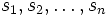Artin braid group

Definition

A group$G$ is termed an Artin braid group if it can be equipped with a finite presentation as follows. The generators are$s_1, s_2, \dots, s_n$ for some$n$, and for every$i \ne j$, we have a nonnegative integer$m_{ij}$, possibly zero, and such that$m_{ij} = m_{ji}$. The relation is given by:$s_is_js_i \dots = s_js_i \dots$

Both sides have length$m_{ij}$. When$m_{ij}$ is odd, the left side ends in$s_i$ and the right side ends in$s_j$. When$m_{ij}$ is even, the left side ends in$s_j$ and the right side ends in$s_i$.

Such a presentation is termed an Artin presentation or Artin braid presentation.

The standard braid group is an example of an Artin braid group, where$m_{ij} = m_{ji} = 3$ for all$|i - j| = 1$ and$m_{ij} = m_{ji} = 2$ for$|i - j| > 1$..

Relation with Coxeter groups

Given a group with an Artin presentation, we can consider a corresponding Coxeter group. The Coxeter group is the quotient of the group by the normal closure of the squares of the generators. In other words it is the quotient by the group$\langle s_i^2 \rangle$. The Coxeter presentation for the Coxeter group obtained has the same$m_{ij}$ as the original group.